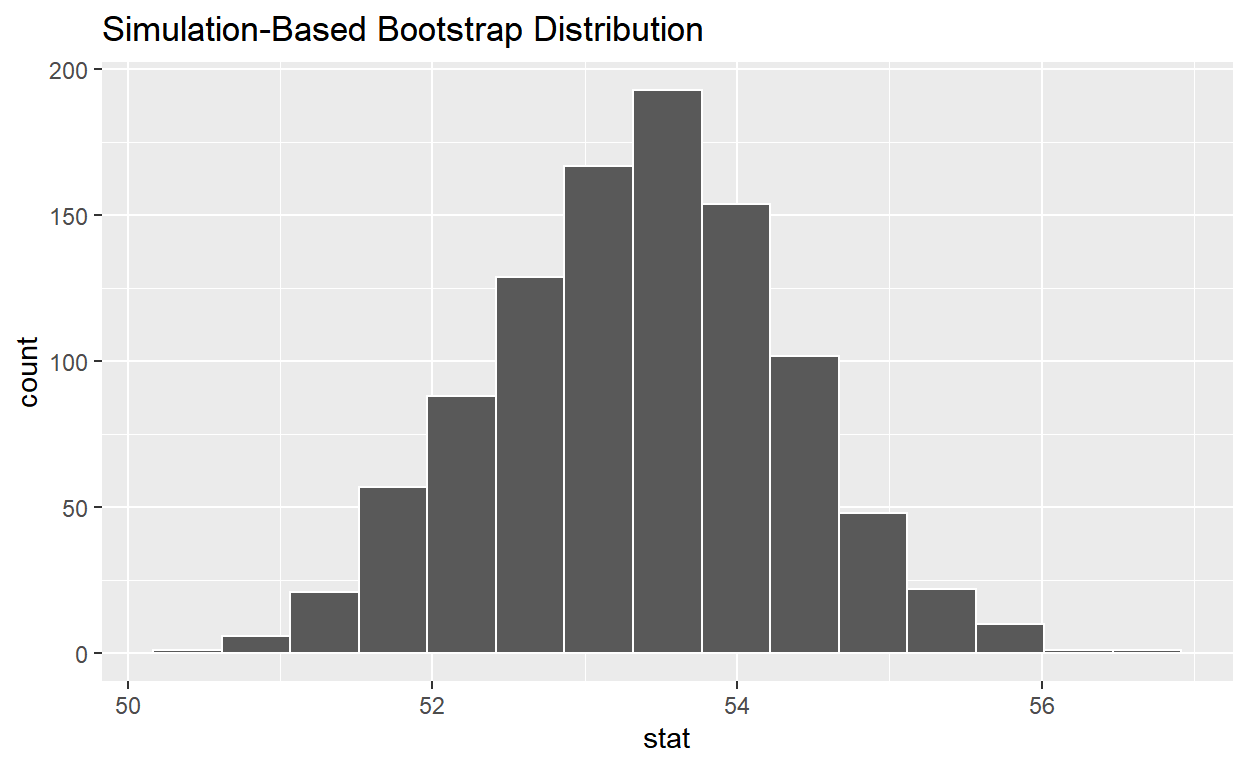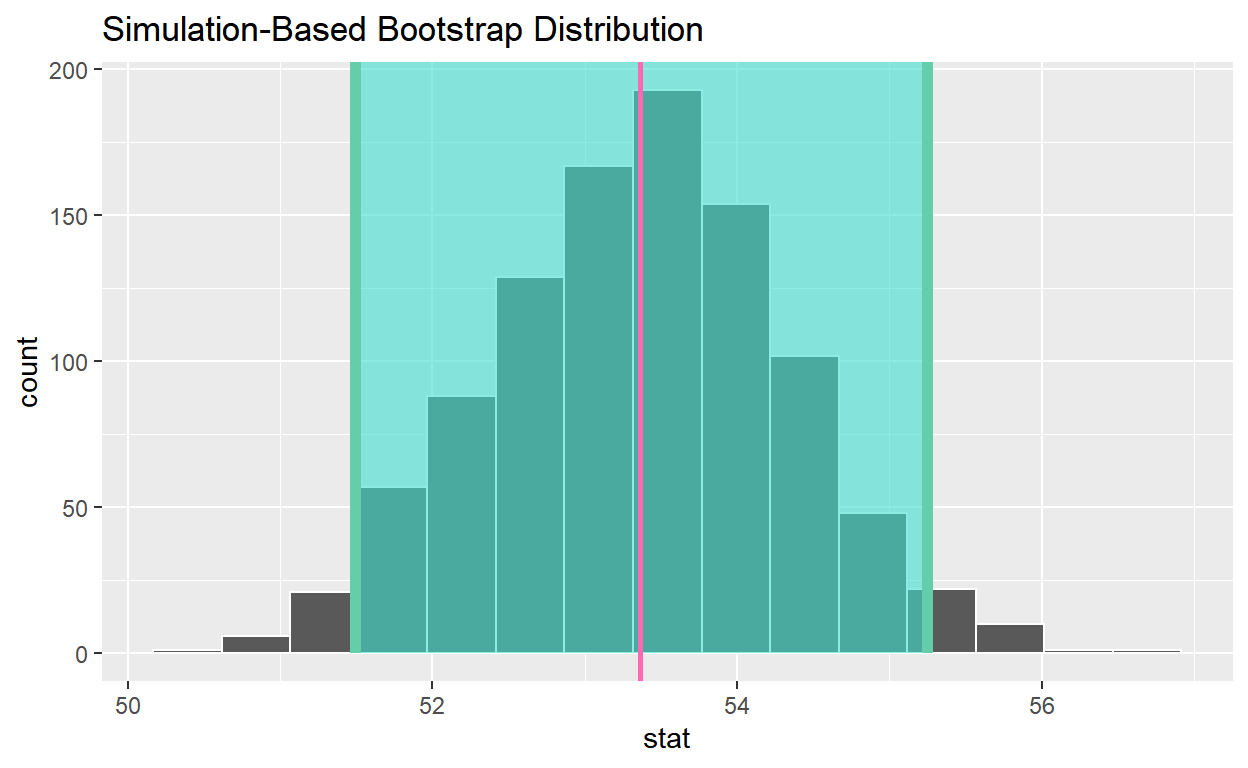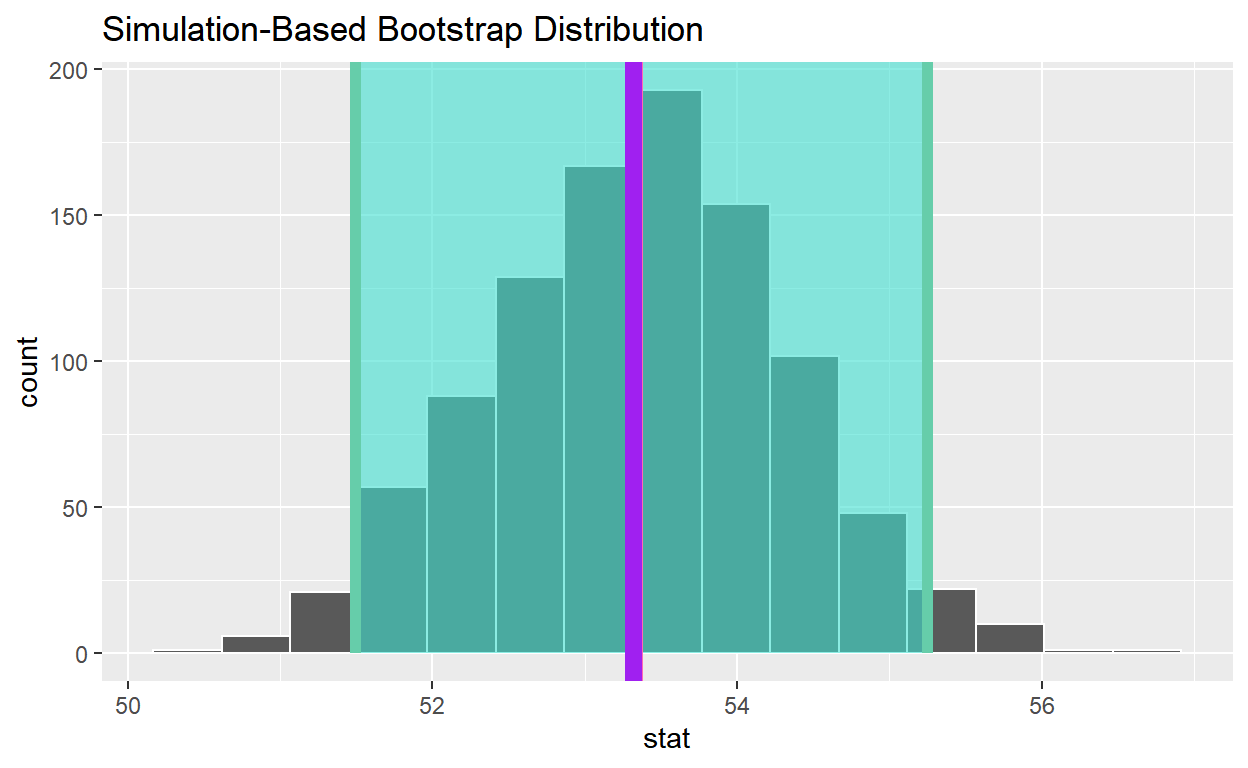# Bootstrapping

Chapter 8 of Moderndive. Code for quiz 12.

Load the R packages we will use.

``````library(tidyverse)
library(moderndive)
library(infer)
library(fivethirtyeight)
``````

What is the average age of members that have served in congress?

• Set random seed generator to 123

• Take a sample of 100 from the dataset `congress_age` and assign it to `congress_age_100`

``````set.seed(123)

congress_age_100 <- congress_age %>%
rep_sample_n(size = 100)
``````
• `congress_age` is the population and `congres_age_100` is the sample.

• `18,635` is the number of observations in the population and `100` is the number of observations in the sample.

Construct the confidence interval

1. Use `specify` to indicate the variable from congress_age_100 that you are interested in

``````congress_age_100 %>%
specify(response = age)
``````
``````Response: age (numeric)
# A tibble: 100 x 1
age
<dbl>
1  53.1
2  54.9
3  65.3
4  60.1
5  43.8
6  57.9
7  55.3
8  46
9  42.1
10  37
# ... with 90 more rows``````

2. `generate` 1000 replicates of your sample of 100

``````congress_age_100 %>%
specify(response = age) %>%
generate(reps = 1000, type = "bootstrap")
``````
``````Response: age (numeric)
# A tibble: 100,000 x 2
# Groups:   replicate [1,000]
replicate   age
<int> <dbl>
1         1  42.1
2         1  71.2
3         1  45.6
4         1  39.6
5         1  56.8
6         1  71.6
7         1  60.5
8         1  56.4
9         1  43.3
10         1  53.1
# ... with 99,990 more rows``````

The output has `100,000` rows.

3. `calculate` the mean for each replicate

• Assign the `bootstrap_distribution_mean_age`

• Display `bootstrap_distribution_mean_age`

``````bootstrap_distribution_mean_age <- congress_age_100 %>%
specify(response = age) %>%
generate(reps = 1000, type = "bootstrap") %>%
calculate(stat = "mean")

bootstrap_distribution_mean_age
``````
``````Response: age (numeric)
# A tibble: 1,000 x 2
replicate  stat
<int> <dbl>
1         1  53.6
2         2  53.2
3         3  52.8
4         4  51.5
5         5  53.0
6         6  54.2
7         7  52.0
8         8  52.8
9         9  53.8
10        10  52.4
# ... with 990 more rows``````

The `bootstrap_distribution_mean_age` has 1000 means.

4. `visualize` the bootstrap distribution

``````visualize(bootstrap_distribution_mean_age)
``````Calculate the 95% confidence interval using the percentile method

• Assign the output to `congress_ci_percentile`

• Display `congress_ci_percentile`

``````congress_ci_percentile <- bootstrap_distribution_mean_age %>%
get_confidence_interval(type = "percentile", level = 0.95)

congress_ci_percentile
``````
``````# A tibble: 1 x 2
lower_ci upper_ci
<dbl>    <dbl>
1     51.5     55.2``````

Calculate the observed point estimate of the mean and assign it to `obs_mean_age`

• Display `obs_mean_age`
``````obs_mean_age <- congress_age_100 %>%
specify(response = age) %>%
calculate(stat = "mean") %>%
pull()

obs_mean_age
``````
`` 53.36``

• Add a line at the observed mean, `obs_mean_age`, to your visualization and color it “hotpink”

``````visualize(bootstrap_distribution_mean_age) +
geom_vline(xintercept = obs_mean_age, color = "hotpink", size = 1)
``````• Calculate the population mean to see if it is in the 95% confidence interval

• Assign the output to `pop_mean_age`

• Display `pop_mean_age`

``````pop_mean_age <- congress_age %>%
summarize(pop_mean = mean(age)) %>% pull()

pop_mean_age
``````
`` 53.31373``
• Add a line to the visualization at the population mean of `pop_mean_age` to the plot, color it “purple”
``````visualize(bootstrap_distribution_mean_age) +
geom_vline(xintercept = obs_mean_age, color = "hotpink", size = 1) +
geom_vline(xintercept = pop_mean_age, color = "purple", size = 3)
````````````ggsave(filename = "preview.png", path = here::here("_posts", "2022-04-22-bootstrapping"))
``````
• Is population mean the 95% confidence interval constructed using the bootstrap distribution?

`Yes`

• Change `set.seed (123)` to `set.seed(4346)`, rerun all the code.

• When you change the seed is the population mean in the 95% confidence interval constructed using the bootstrap distribution?

`No`

• If you construct 100 95% confidence intervals approximately how many do you expect will contain the population mean?

`95`